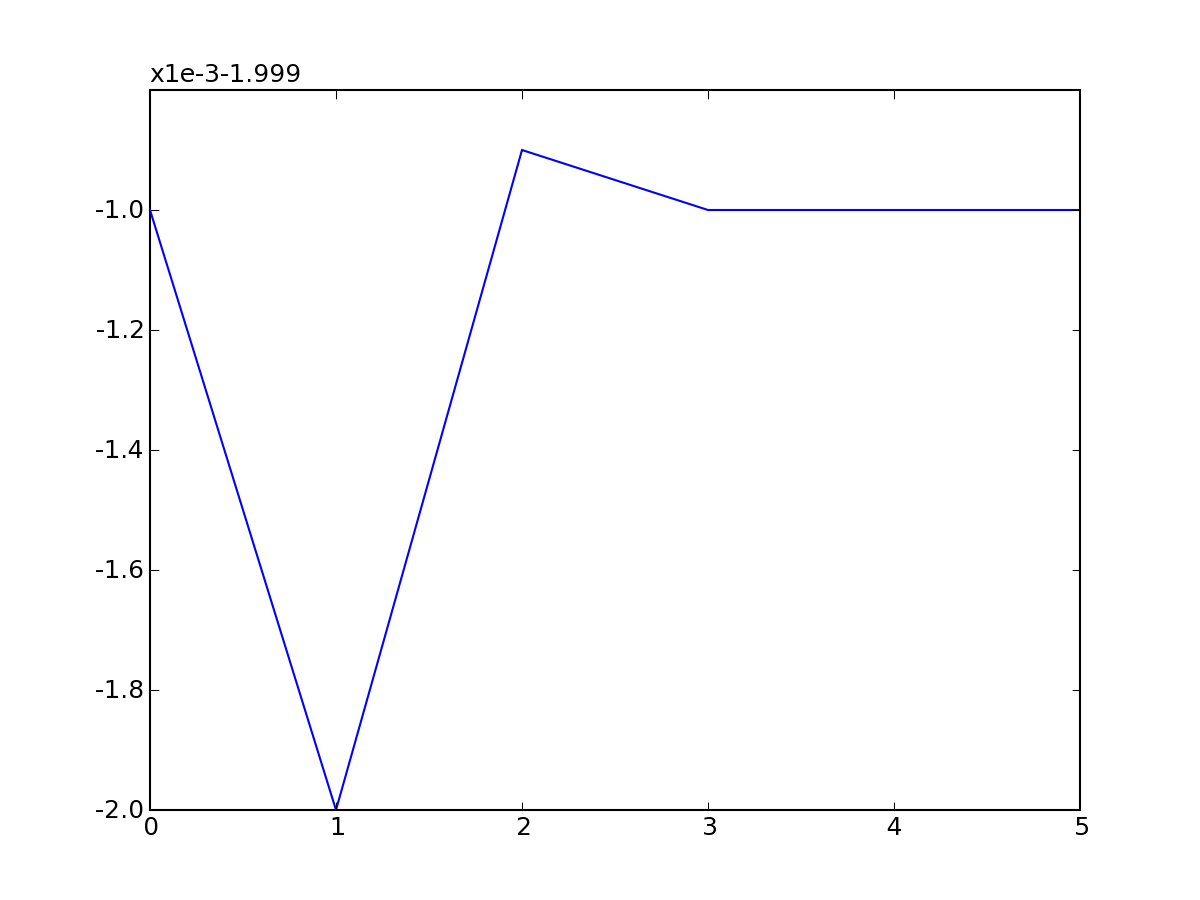# Weird zoom when plotting an almost constant line

Hello,

When mpl plots equal values, it produces a line as expected. But if the values are equal C +/- epson, with epson <= 0.001 where C is a constant then the vertical range is presented as (for example) x1e-3-1.999 which is not at all clear. Here's an example (png attached):

#! /usr/bin/python
from pylab import *

x = [0.0,1.0,2.0,3.0,4.0,5.0]
y= [-2.0,-2.001,-1.9999,-2.0,-2.0,-2.0]

plot(x,y)
show()

Is there a way to limit the maximum default zoom? Although the example uses pylab I'm really using mpl OO interface (on a linux machine).

Thanks,

Jo�o Lu�s Silvareplying to myself, but I found a workaround

int = ax.yaxis.get_view_interval()
ymin,ymax = int.get_bounds()
if ymax - ymin < 0.01:
ymin = ymin - 0.005
ymax = ymax + 0.005
int.set_bounds(ymin,ymax)

Is there a better way?